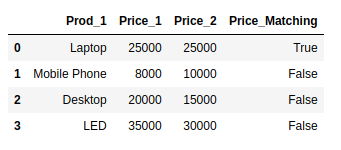# How to compare values in two Pandas Dataframes?

• Last Updated : 12 Jan, 2022

Let’s discuss how to compare values in the Pandas dataframe. Here are the steps for comparing values in two pandas Dataframes:

Step 1 Dataframe Creation: The dataframes for the two datasets can be created using the following code:

## Python3

 `import` `pandas as pd` `# elements of first dataset``first_Set ``=` `{``'Prod_1'``: [``'Laptop'``, ``'Mobile Phone'``,``                        ``'Desktop'``, ``'LED'``],``             ``'Price_1'``: [``25000``, ``8000``, ``20000``, ``35000``]``             ``}` `# creation of Dataframe 1``df1 ``=` `pd.DataFrame(first_Set, columns``=``[``'Prod_1'``, ``'Price_1'``])``print``(df1)` `# elements of second dataset``second_Set ``=` `{``'Prod_2'``: [``'Laptop'``, ``'Mobile Phone'``,``                         ``'Desktop'``, ``'LED'``],``              ``'Price_2'``: [``25000``, ``10000``, ``15000``, ``30000``]``              ``}` `# creation of Dataframe 2``df2 ``=` `pd.DataFrame(second_Set, columns``=``[``'Prod_2'``, ``'Price_2'``])``print``(df2)`

Output:Step 2 Comparison of values: You need to import numpy for the successful execution of this step. Here is the general template to perform the comparison:

df1[‘new column for the comparison results’] = np.where(condition, ‘value if true’, ‘value if false’)

Example: After execution of this code, the new column with the name Price_Matching will be formed under df1. Columns result will be displayed according to the following conditions:

• If Price_1 is equal to Price_2, then assign the value of True
• Otherwise, assign the value of False.

## Python3

 `import` `numpy as np` `# add the Price2 column from``# df2 to df1``df1[``'Price_2'``] ``=` `df2[``'Price_2'``]` `# create new column in df1 to``# check if prices match``df1[``'Price_Matching'``] ``=` `np.where(df1[``'Price_1'``] ``=``=` `df2[``'Price_2'``],``                                 ``'True'``, ``'False'``) ``df1`

Output:My Personal Notes arrow_drop_up